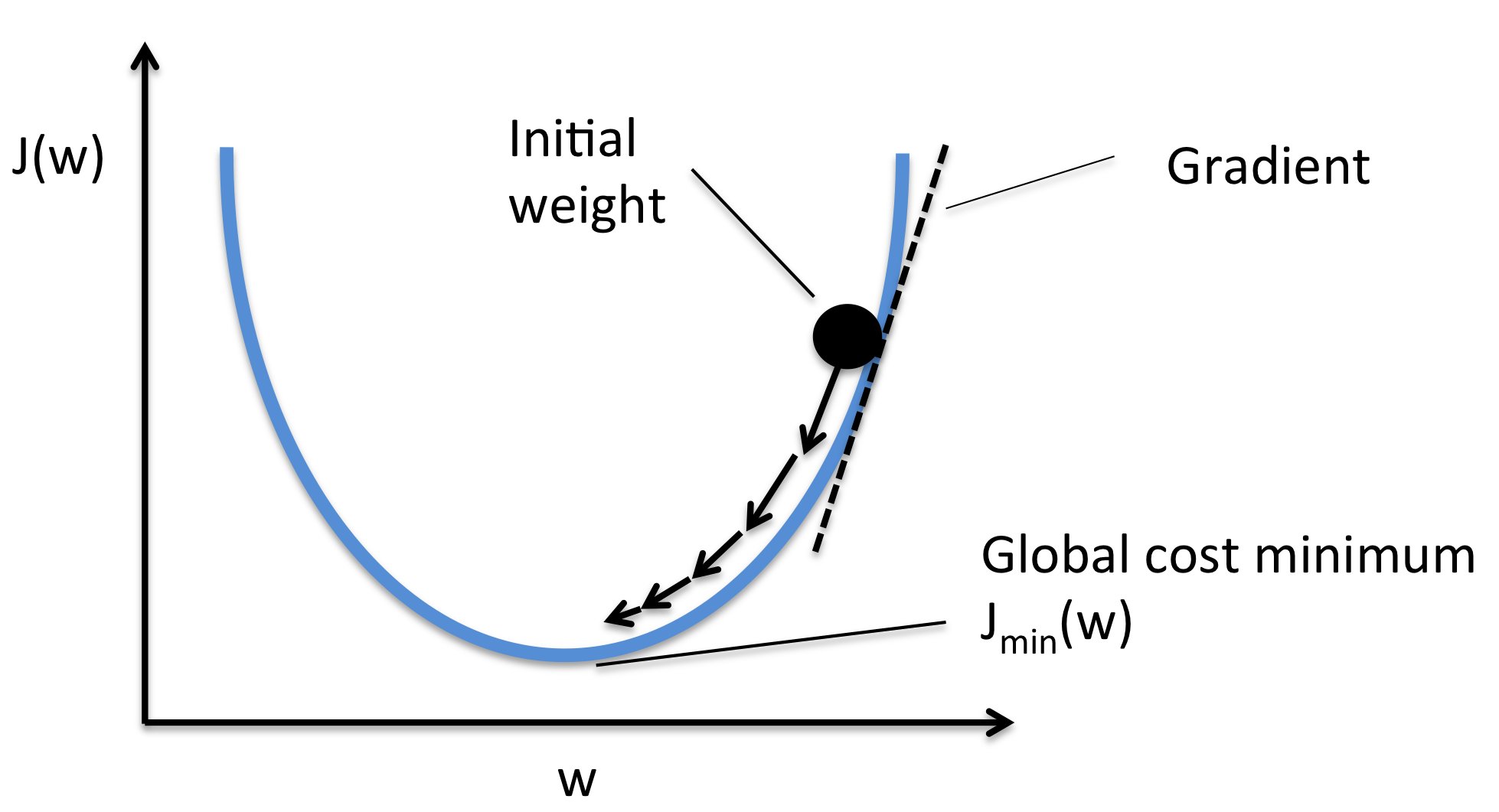## Optimization

• How we can optimize the loss functions we discussed?
• Strategy one:
• Generate random parameters and try all of them on the loss and get the best loss in a brute force fashion. Highly inefficient.
• Strategy two:
• Follow the slope.• Our goal is to compute the gradient of each parameter we have.
• Numerical gradient: Approximate, slow, easy to write. (But its useful in debugging.)
• Analytic gradient: Exact, Fast, Error-prone. (Always used in practice)
• After we compute the gradient of our parameters, we compute the gradient descent:

$W = W - \alpha * W_{grad}$
• where $$\alpha$$ is the learning rate and $$W_{grad}$$ is the gradient of the weights w.r.t. the parameters.
• learning_rate is so important hyper parameter you should get the best value of it first of all the hyperparameters.

• Instead of using all the date, use a mini batch of examples (32/64/128 are commonly used) for faster results.

## Citation

If you found our work useful, please cite it as:

@article{Chadha2020IntroductionToCNNsforVisualRecognition,
title   = {Introduction to CNNs for Visual Recognition},